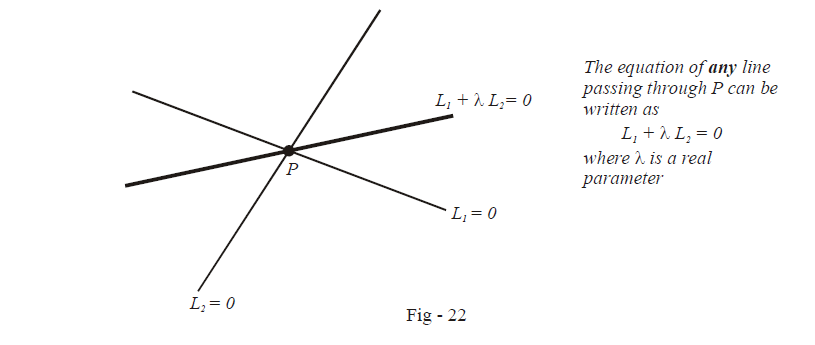# Examples on Concurrency of Three Lines Set 2

Go back to  'Straight Lines'

Example – 11

Show that the equation of any line passing through the intersection point P of two given lines whose equations are L1 and L2, can be expressed as $${L_1} + \lambda \,{L_2} = 0,\,\,{\rm{where}}\,\,\lambda$$ is a real parameter.

Solution: Let $${L_1} \equiv {a_1}x + {b_1}y + {c_1} = 0$$ and $${L_2} \equiv {a_2}x + {b_2}y + {c_2} = 0$$

Consider the equation $${L_1} + \lambda \,{L_2} = 0 \qquad \qquad \qquad \qquad \qquad \qquad \dots\rm{(1)}$$

\begin{align} \Rightarrow & \qquad {a_1}x + {b_1}y + {c_1} + \lambda \left( {{a_2}x + {b_2}y + {c_2}} \right) = 0\\ \Rightarrow & \qquad \left( {{a_1} + \lambda {a_2}} \right)x + \left( {{b_1} + \lambda {b_2}} \right)y + \left( {{c_1} + \lambda {c_2}} \right) = 0 \end{align}

This is definitely the equation of a straight line because it is of the form $$ax + by + c = 0.$$ Also, notice in addition that the intersection point P will satisfy this equation, because if we substitute the co-ordinates of the intersection point P in (1), both L1 and L2 vanish.

Thus, $${L_1} + \lambda {L_2} = 0$$ is the equation of an arbitrary straight line that passes through the intersection point P of L1 and L2. (As we vary $$\lambda ,$$ the slope of this line will vary but it will always pass through P).This result is very beneficial in certain cases. We’ll see such cases in some subsequent examples

Example – 12

Find the equations to the straight lines passing through

(a) (3, 2) and the point of intersection of $$2x + 3y = 1 \text{ and } 3x - 4y = 6$$

(b) Origin and the point of intersection of \begin{align}\frac{x}{a} + \frac{y}{b} = 1{\text{ and }} \frac{x}{b} + \frac{y}{a} = 1\end{align}.

Solution:(a) The equations of the two given lines in standard form are :

\begin{align}&{L_1}\,\,\,\, \equiv \,\,\,2x + 3y - 1 = 0\\&{L_2}\,\,\,\, \equiv \,\,\,3x - 4y - 6 = 0\end{align}

Any line passing through the intersection point of L1 and L2 is

\begin{align}&{L_1} + \lambda {L_2} = 0\\\Rightarrow \qquad &(2x + 3y - 1) + \lambda (3x - 4y - 6) = 0\\\Rightarrow \qquad &(2 + 3\lambda )x + (3 - 4\lambda )y - (1 + 6\lambda ) = 0 \qquad \qquad\qquad \dots \rm{(1)} \end{align}

We want this line to pass through (3, 2). Therefore (3, 2) must satisfy the equation of this line, i.e.

\begin{align}&(2 + 3\lambda )3 + (3 - 4\lambda )2 - (1 + 6\lambda ) = 0\\\Rightarrow \quad &- 5\lambda + 11 = 0\\\Rightarrow \quad &\lambda = \frac{{11}}{5}\end{align}

We substitute \begin{align}\lambda = \frac{{11}}{5}\end{align} in (1) to get the required equation:

\begin{align}&(2 + 2 \cdot \frac{{11}}{5})x + (3 - 4 \cdot \frac{{11}}{5})y - (1 + 6 \cdot \frac{{11}}{5}) = 0\\\Rightarrow \qquad &43x - 29y - 71 = 0\end{align}

(b) We follow the same procedure as in part (a)

\begin{align}{L_1}\,\,\,\,:\,\,\,bx + ay - ab = 0\\{L_2}\,\,\,\,:\,\,\,ax + by - ab = 0\end{align}

The equation of any line passing through the intersection point of L1 and L2 is

\begin{align}&{L_1} + \lambda {L_2} = 0\\\Rightarrow \qquad &(b + \lambda a)x + (a + \lambda b)y - ab(1 + \lambda ) = 0 \qquad \qquad \dots \rm{(2)}\end{align}

Since this line must pass through (0, 0), we substitute (0, 0) into (2) to get

\begin{align}&ab(1 + \lambda ) = 0\\\Rightarrow \qquad &\lambda = - 1\end{align}

We substitute $$\lambda = - 1$$ into (2) to get the required equation :

\begin{align}&(b - a)x + (a - b)y = 0\\\Rightarrow \qquad &x - y = 0\end{align}

Straight Lines
Straight Lines
grade 11 | Questions Set 1
Straight Lines
Straight Lines
grade 11 | Questions Set 2